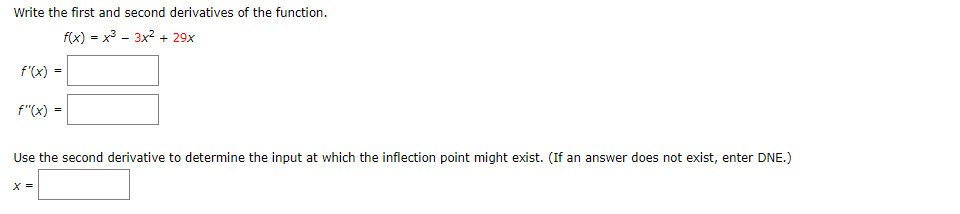# Write the first and second derivatives of the functionf(x) = x3 - 3x2 29xf'(x)f"(x) =Use the second derivative to determine the input at which the inflection point might exist. (If an answer does not exist, enter DNE.)

Question
1 viewshelp_outlineImage TranscriptioncloseWrite the first and second derivatives of the function f(x) = x3 - 3x2 29x f'(x) f"(x) = Use the second derivative to determine the input at which the inflection point might exist. (If an answer does not exist, enter DNE.) fullscreen
check_circle

Step 1

Given function is

Step 2

Using formula

Step 3

The first derivative ...

### Want to see the full answer?

See Solution

#### Want to see this answer and more?

Solutions are written by subject experts who are available 24/7. Questions are typically answered within 1 hour.*

See Solution
*Response times may vary by subject and question.
Tagged in

### Calculus